# Precalculus : Parallel and Perpendicular Vectors in Two Dimensions

## Example Questions

1 2 4 Next →

### Example Question #31 : Determine If Two Vectors Are Parallel Or Perpendicular

Find the angle between the vectors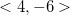and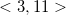.

Possible Answers: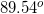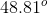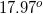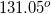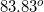Correct answer:Explanation:

To determine the angle between our two vectors, we can use the fact that for any 2 vectors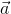and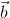,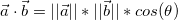where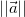is the magnitude and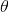is the angle between the 2 vectors, which is what we are looking for.

Working from the left, we can first find the dot product,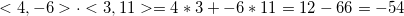Now we'll find the magnitudes of the two vectors by using the Pythagorean Theorem: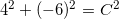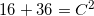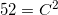take the square root of both sides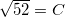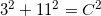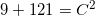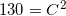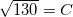Now we can plug these values back into the equation to start solving for theta: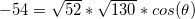multiply the two numbers inside the radicals: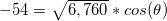divide both sides by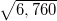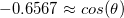take the inverse cosine of both sides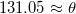### Example Question #32 : Determine If Two Vectors Are Parallel Or Perpendicular

Find the angle between the vectors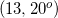and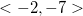. Note that the first vector is in polar form and the second is in component form.

Possible Answers: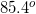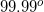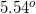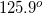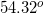Correct answer:Explanation:

To find the angle between two vectors, we can use the fact that. In order to find the dot product, we need to convert the vectorto component form. This is easiest to do after drawing a quick sketch of the vector: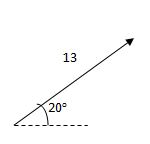To find the vertical component, set up an equation involving sine, since the vertical component is the side of a right triangle across from the 20-degree angle: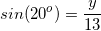evaluate the sine of 20, then multiply by 13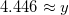To find the horizontal component, set up an equation involving cosine, since the horizontal component is the side of a right triangle adjacent to the 20-degree angle: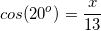evaluate the cosine of 20, then multiply by 13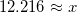The component form of this vector can be written as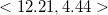Next, we can find the magnitude of the vector already in component form by using the Pythagorean Theorem: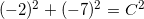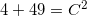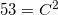take the square root of both sides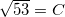Now we have all the information that we need to solve for theta.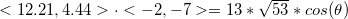Find the dot product for the left side: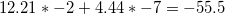which we can now put back in the equation: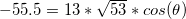now divide both sides by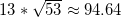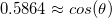take invese cosine of both sides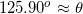### Example Question #33 : Determine If Two Vectors Are Parallel Or Perpendicular

Find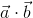where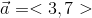and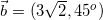. Note thatis in component form whileis in polar form.

Possible Answers: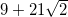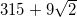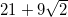Correct answer:Explanation:

First, convertto component form. The easiest way to do that would be with special right triangles, knowing that for a 45-45-90 triangle the ratio of the hypotenuse to both of the legs is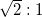. So if the magnitude of the vector is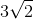, then the components must both be 3.

This could also be found by setting up and solving the equations: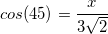and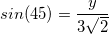Now that both vectors are component form, we see that we're multiplying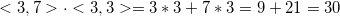### Example Question #34 : Determine If Two Vectors Are Parallel Or Perpendicular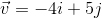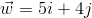Which of the following best explains whether the two vectors above are perpendicular or parallel?

Possible Answers:

Neither perpendicular nor parallel, because their dot product is neither zero nor one.

Perpendicular, because their dot product is zero.

Perpendicular, because their dot product is one.

Parallel, because their dot product is one.

Parallel, because their dot product is zero.

Correct answer:

Perpendicular, because their dot product is zero.

Explanation:

Two vectors are perpendicular if their dot product is zero, and parallel if their dot product is 1.

Take the dot product of our two vectors to find the answer: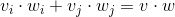Using our given vectors: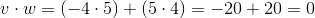Thus our two vectors are perpendicular.

1 2 4 Next →

### All Precalculus Resources